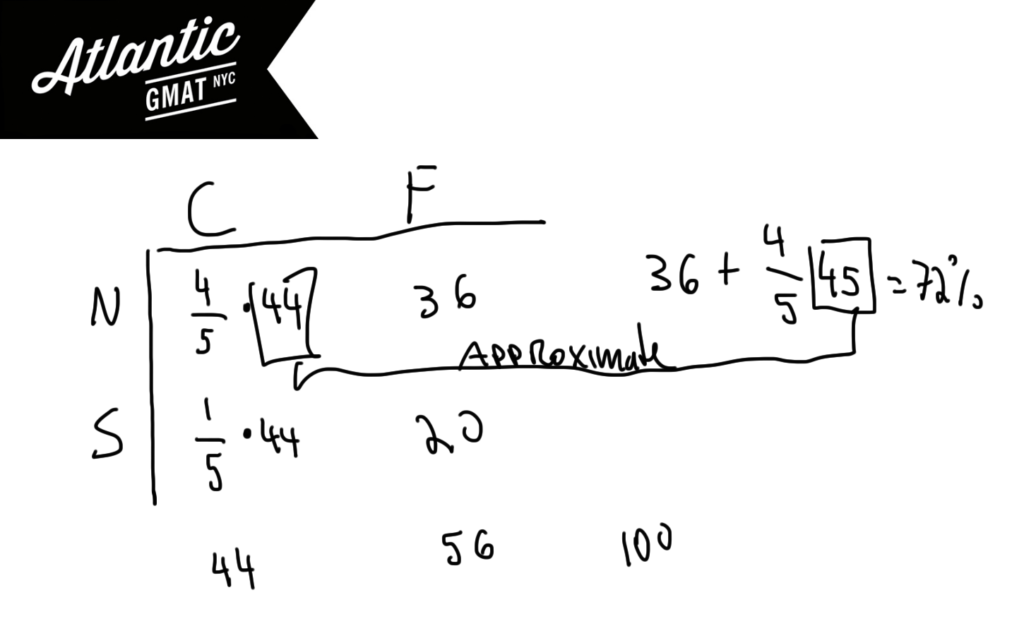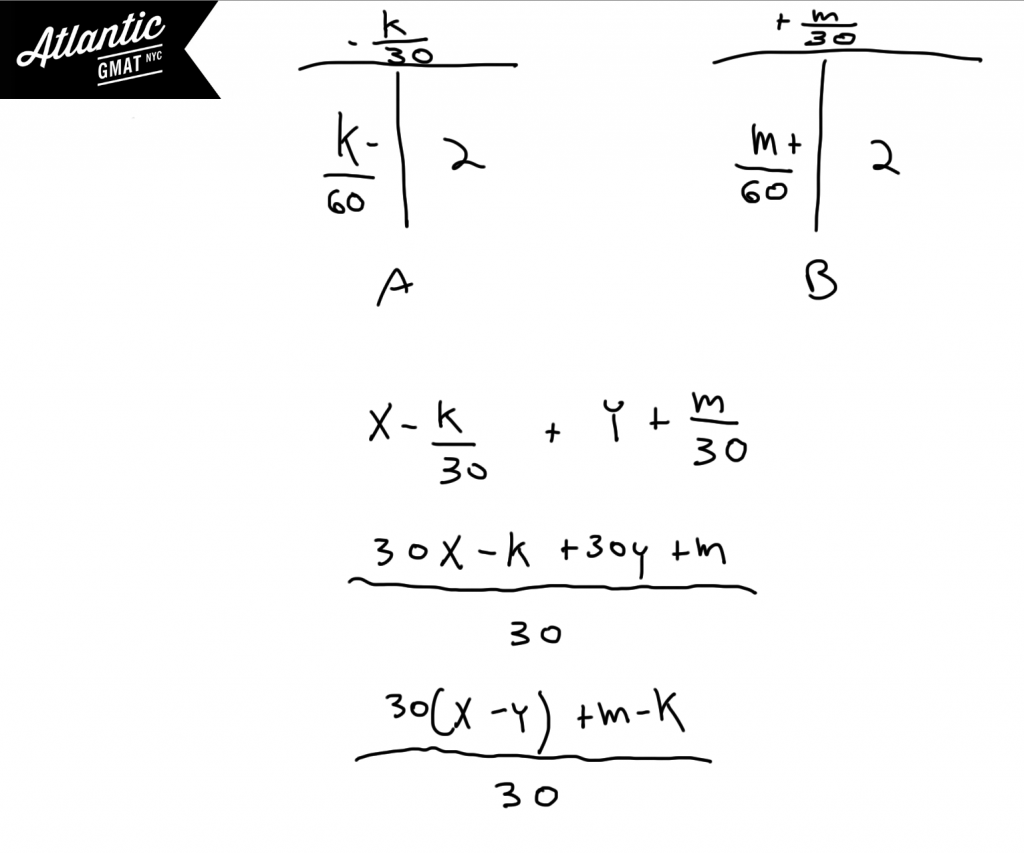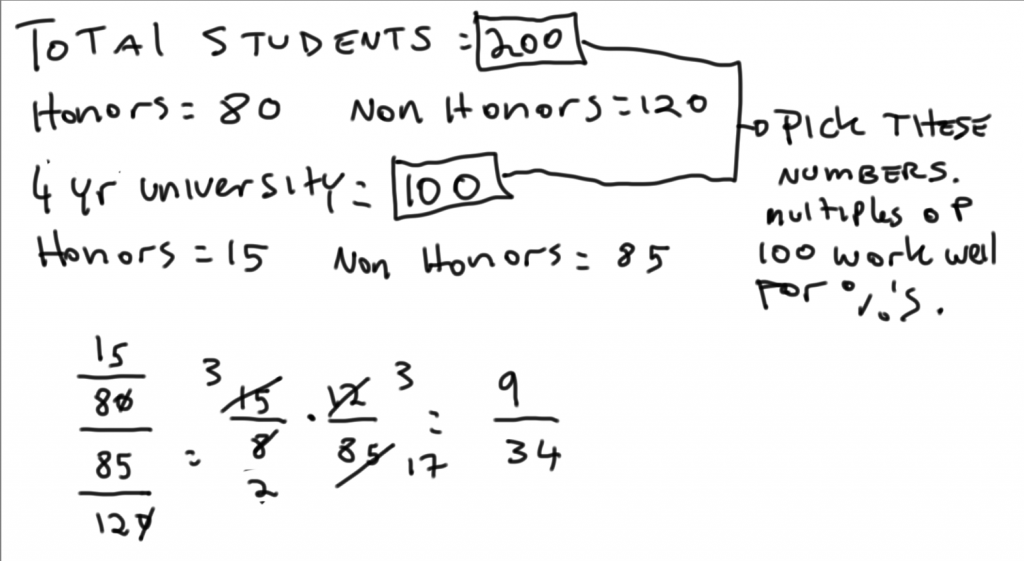Private GMAT Tutoring in NYC & Online

# GMAT Question of the Day - Problem Solving - Overlapping Sets/Picking Numbers

At a certain rail-yard each train is either a freight train or a commuter train and either runs on the southern line or the northern line. 20% of the commuter trains run on the southern line and the number of freight trains that run on the southern line represents 20% of the total trains at the rail yard. If the commuter trains represent 44% of the total trains at the rail yard, approximately what percent of the trains run on the northern line?

A. 41%

B. 46%

C. 57%

D. 63%

E. 71%

## GMAT Question of the Day Solution

These GMAT overlapping sets questions are straightforward but take some organizing so it's important to take your time with the set-up. On the whole, the GMAT is an organizational challenge. Try picking a value for the total. That should make things easier. Whenever you are dealing with a question that has variables or percents/proportions in the answer choices consider picking numbers to make the question more concrete. Also, did you define what you were looking for: Northern/Total? Defining the question will help you plan your work and will draw you towards the solution.# GMAT Question of the Day - Problem Solving - Work

Tank A contains X gallons of water while Tank B contains Y gallons. Tank A has water removed at a rate of K gallons per hour and Tank B has water added at a rate of M gallons per hour. Assuming that both tanks still contain some water, in terms of X, Y, K, and M how many gallons of water will both tanks have 2 minutes from now?

A.  (x - y - m + k)/60

B. (30(x-y) - m + k)/60

C. (30(x-y) + m - k)/60

D. (30(x-y) + m - k)/30

E. (30(x-y) - m + k)/30

## GMAT Question of the Day Solution

For GMAT work and rate questions it helps to organize the information in T's. This way you have a place to store the numbers in a way that identifies what the numbers represent. The T's also help you make inferences. Notice that in this question there is a unit conversion from minutes to hours. For GMAT work and rate questions it's a great idea to double check the units before committing to an answer.# GMAT Question of the Day - Problem Solving - Ratio

A total of 40% of the students who participated in an academic survey of high school students were graduating with honors. 15% of the students who said that they planned to attend a 4 year university were graduating with honors. What is the ratio of the percent of honors students who plan to attend a 4 year university to the percent of non-honors students who plan to attend a 4 year university?

A. 9/34

B. 11/34

C. 15/37

D. 16/37

E. 18/37

## GMAT Question of the Day Solution:

This is a great candidate for picking numbers. Why? You are given a bunch of proportions in the question and then the answers are also in proportions. So you can set the value for how many students you have in total and how many plan on attending a 4 year university. After the numbers are set filling in the details should be relatively simple. When dealing with percents it helps to pick numbers that are multiples of 100. Also - remember to simplify as much as possible before doing any multiplication. The GMAT tends to give opportunities to simplify any labor intensive calculations so it's a good idea to pause before making any big moves.# CONTACT

Atlantic GMAT

405 East 51st St.

NY, NY 10022

(347) 669-3545

info@AtlanticGMAT.com